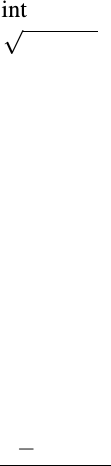Study Guides (390,000)
US (220,000)
UC-Irvine (3,000)
MATH (600)
MATH 2B (100)
All (60)
Midterm

# MATH 2B Study Guide - Midterm Guide: AntiderivativeExam

Department
Mathematics
Course Code
MATH 2B
Professor
All
Study Guide
Midterm

This preview shows page 1. to view the full 5 pages of the document.
MATH 2B: SAMPLE MIDTERM #1
This exam consists of 5 questions and 85 total points.
Read the directions for each problem carefully and answer all parts of each problem.
Please show all work needed to arrive at your solutions (unless instructed otherwise). Label
graphs and deﬁne any notation used. Cross out incorrect scratch-work.
No calculators or other forms of assistance are allowed. Do not check your cell phones
during the exam.
1. (15 points)
a. Estimate the area under the graph of f(x) = x2+xfrom x= 0 to x= 3 using 3 approxi-
mating rectangles and left endpoints.
b. Estimate the area under the graph of f(x) = x1from x= 0 to x= 6 using 3 rectangles
and midpoints.
c. Find an expression for the area under the graph of f(x) = x2+xfrom x= 2 to x= 5 as a
limit of Riemann sums. (You do not need to evaluate the limit.)

Unlock to view full version

Only page 1 are available for preview. Some parts have been intentionally blurred.2. (15 points) Evaluate each of the following indeﬁnite integrals.
a. Rx3x21dx
b. Z1sin2(x)
cos xdx
c. Rsin(7θ+ 5)